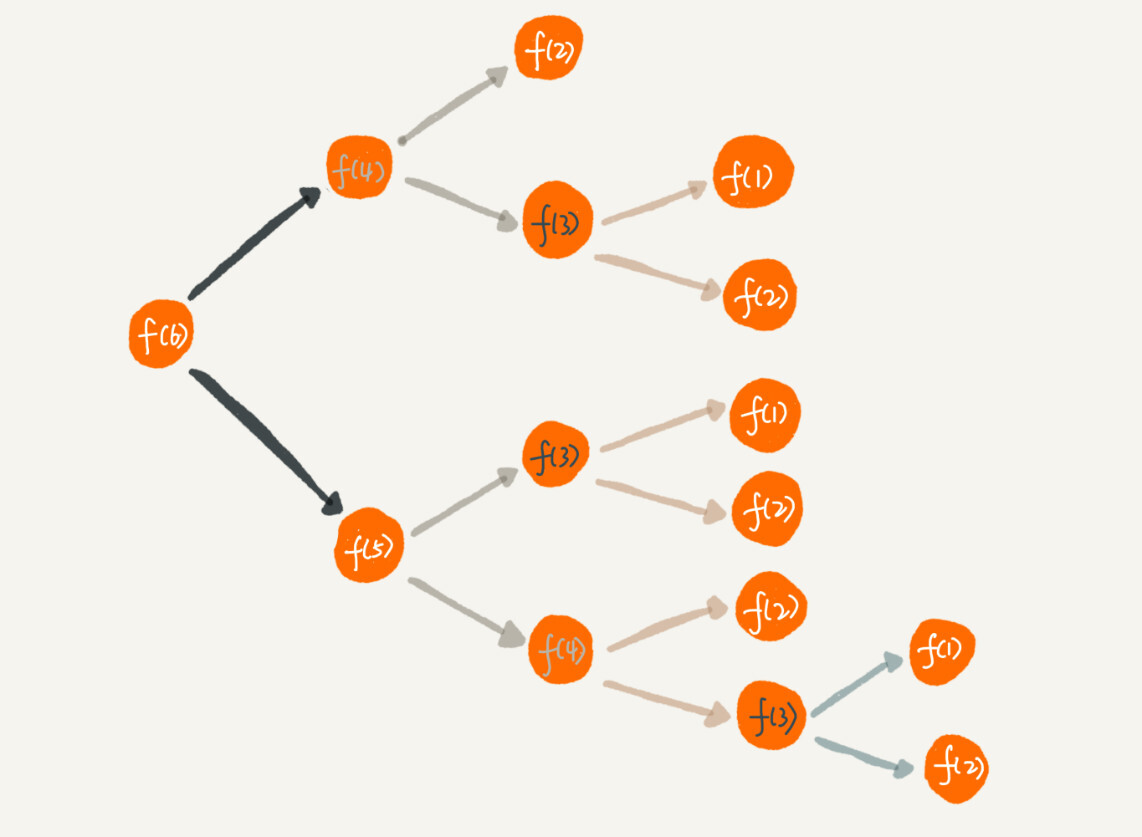# 数据结构与算法之美学习笔记

## 基础篇之递归

Posted by Alee on October 9, 2019

## 基础篇之递归

#### 如何理解递归

``````f(n) = f(n-1) + 1 其中 f(1) = 1
``````

f(n)表示想知道自己在哪一排，f(n-1)表示前面一排所在的排数，f(1) = 1表示第一排的人知道自己在第一排。有了这个递推公式，我们就可以很轻松地将它改为递归代码：

``````int f(int n) {
if (n == 1) return 1;
return f(n - 1) + 1;
}
``````

#### 递归需要满足的三个条件

1. 一个问题的解可以分解为几个子问题的解

何为子问题？子问题就是数据规模更小的问题。比如前面的案例，要知道“自己在哪一排”，可以分解为“前一排的人在哪一排”这样的一个子问题。

2. 这个问题与分解之后的子问题，除了数据规模不同，求解思路完全一样

如案例所示，求解“自己在哪一排”的思路，和前面一排人求解“自己在哪一排”的思路是一模一样的。

3. 存在递归终止条件

把问题分解为子问题，把子问题再分解为子子问题，一层一层分解下去，不能存在无限循环，这就需要有终止条件。前面的案例：第一排的人知道自己在哪一排，不需要再问别人，f(1) = 1就是递归的终止条件。

#### 怎样编写递归代码

``````f(n) = f(n-1) + f(n-2)
``````

n = 2时，f(2) = f(1) + f(0)。如果递归终止条件只有一个f(1) = 1，那f(2)就无法求解了。所以除了f(1) = 1这一个递归终止条件外，还要有f(0) = 1，表示走0个台阶有一种走法，不过这样看起来不符合正常的逻辑思维。所以，我们可以把f(2) = 2作为一种终止条件，表示走2个台阶，只有两种走法，一步走完或者分两步走。

``````f(1) = 1;
f(2) = 2;
f(n) = f(n - 1) + f(n - 2);
``````

``````int f(int n) {
if (n == 1) return 1;
if (n == 2) return 2;
return f(n -1) + f(n - 2);
}
``````

#### 递归代码要警惕堆栈溢出

``````// 全局变量，表示递归的深度。
int depth = 0;

int f(int n) {
++depth;
if (depth > 1000) throw exception;

if (n == 1) return 1;
return f(n-1) + 1;
}
``````

#### 递归代码要警惕重复计算``````public int f(int n) {
if (n == 1) return 1;
if (n == 2) return 2;

// hasSolvedList 可以理解成一个 Map，key 是 n，value 是 f(n)
if (hasSolvedList.containsKey(n)) {
return hasSovledList.get(n);
}

int ret = f(n-1) + f(n-2);
hasSovledList.put(n, ret);
return ret;
}
``````

#### 怎么将递归代码改写为非递归代码

``````//案例一
int f(int n) {
int ret = 1;
for (int i = 2; i <= n; ++i) {
ret = ret + 1;
}
return ret;
}
``````
``````//案例二
int f(int n) {
if (n == 1) return 1;
if (n == 2) return 2;

int ret = 0;
int pre = 2;
int prepre = 1;
for (int i = 3; i <= n; ++i) {
ret = pre + prepre;
prepre = pre;
pre = ret;
}
return ret;
}
``````

#### 解答开篇

``````long findRootReferrerId(long actorId) {
Long referrerId = select referrer_id from [table] where actor_id = actorId;
if (referrerId == null) return actorId;
return findRootReferrerId(referrerId);
}
``````

1. 如果递归很深，可能会有堆栈溢出的问题。

2. 如果数据库存在脏数据，可能会出现无限递归的问题。

比如demo环境下数据库中，测试工程师为了方便测试，会人为地插入一些数据，就会出现脏数据。如果A的推荐人是B，B的推荐人是C，C的推荐人是A，这样就会发生死循环。

#### 思考

1. 调试递归：1.打印日志发现递归值。2.结合条件断点进行调试。